## Wednesday, August 30, 2017

### Pearson correlation with phylogenetic data

Today I was asked the following question:

“I am interested in testing the correlation between 2 traits taking into account for the phylogenetic structure in my dataset. PGLS is a good way to test it, but I was wondering if a simple correlation test (using a Pearson r) would be possible. Your `phyl.vcv` computes a phylogenetic trait variance-covariance matrix between two variables. Can I use this matrix to compute a phylogenetic Pearson r value? If so, can this r-value be used to compute the statistic to test the significance of the correlation (with n-2 df in a t distribution)?”

The question was also posted here.

The answer is basically, yes & yes - but it is not strictly necessary as PGLS will give us the same p-value. Let's see how:

``````library(phytools)
## our data
tree
``````
``````##
## Phylogenetic tree with 26 tips and 25 internal nodes.
##
## Tip labels:
##  A, B, C, D, E, F, ...
##
## Rooted; includes branch lengths.
``````
``````X
``````
``````##              x           y           z
## A -0.723942909  0.96553388  0.50467165
## B -0.034600412  1.48143249  0.66533528
## C -0.001282309  0.62094719  0.60352347
## D -0.100971061  1.23102030  0.01866007
## E -0.363619319  0.06831715  0.91782821
## F -0.400834652 -0.07312087  0.77584466
## G -0.037785409  0.18406320  1.18509956
## H -1.284112703  0.26824899  0.15203366
## I -0.573447373  1.56312743  0.58464867
## J -0.275070942  0.05165640 -0.24120290
## K -1.050699705  0.31715393 -0.63071969
## L -0.689968116  0.22713074 -0.04087866
## M -0.572979102  0.16002629 -0.67389194
## N -0.353661739  0.31157683 -0.60997825
## O -1.720295244  0.57079006 -2.24647340
## P -2.830254149  0.78574612 -2.39094415
## Q -0.104129552  0.93488329  1.66983162
## R -0.324883255  1.00993141 -1.87523279
## S -1.330114250  0.53192888 -2.47716184
## T -0.394635253  0.84544343 -2.05151552
## U -2.788040191  0.75579827 -1.90934711
## V  0.076717481 -1.13500947 -0.01313445
## W  1.388265725 -1.15038331  0.50393587
## X  1.423667629 -1.41685533  1.29662644
## Y  1.459867791 -0.53755989  1.83210421
## Z  2.235835060 -1.28003540  0.84061852
``````

First, let's compute the evolutionary (phylogenetic) correlation between `x` & `y`:

``````## our covariance matrix
obj<-phyl.vcv(X,vcv(tree),1)
obj\$R
``````
``````##            x          y         z
## x  0.6810667 -0.0592844 0.3212055
## y -0.0592844  0.4238996 0.0413417
## z  0.3212055  0.0413417 0.6319725
``````
``````## correlation between x & y
r.xy<-cov2cor(obj\$R)["x","y"]
## t-statistic & P-value
t.xy<-r.xy*sqrt((Ntip(tree)-2)/(1-r.xy^2))
P.xy<-2*pt(abs(t.xy),df=Ntip(tree)-2,lower.tail=F)
P.xy
``````
``````##  0.5915613
``````

As noted before, this is the same P-value as we would obtain fitting a linear model using PGLS of `y~x` or `x~y`.

``````fit.yx<-gls(y~x,data=as.data.frame(X),correlation=corBrownian(1,tree))
anova(fit.yx)
``````
``````## Denom. DF: 24
##             numDF   F-value p-value
## (Intercept)     1 0.2645170  0.6117
## x               1 0.2957732  0.5916
``````
``````fit.xy<-gls(x~y,data=as.data.frame(X),correlation=corBrownian(1,tree))
anova(fit.xy)
``````
``````## Denom. DF: 24
##             numDF   F-value p-value
## (Intercept)     1 0.1812473  0.6741
## y               1 0.2957732  0.5916
``````

Now let's see an example in which a weak correlation exists between the two characters:

``````## correlation between x & z
r.xz<-cov2cor(obj\$R)["x","z"]
## t-statistic & P-value
t.xz<-r.xz*sqrt((Ntip(tree)-2)/(1-r.xz^2))
P.xz<-2*pt(abs(t.xz),df=Ntip(tree)-2,lower.tail=F)
P.xz
``````
``````##  0.01112811
``````
``````fit.zx<-gls(z~x,data=as.data.frame(X),correlation=corBrownian(1,tree))
anova(fit.zx)
``````
``````## Denom. DF: 24
##             numDF  F-value p-value
## (Intercept)     1 0.003181  0.9555
## x               1 7.566719  0.0111
``````
``````## visualize it
phylomorphospace(tree,X[,c("x","z")],node.size=c(0,0))
points(X[,c("x","z")],pch=21,cex=1.5,bg="grey")
text(-3.2,2,paste("evolutionary correlation\nr = ",round(r.xz,4),
", P = ",round(P.xz,4),sep="" ),pos=4)
``````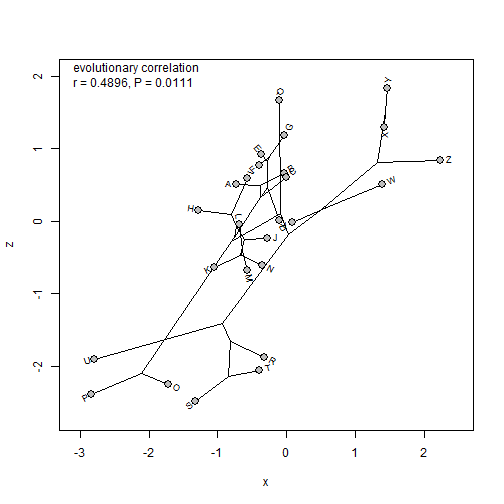Neat.

The data for this example were simulated as follows:

``````tree<-pbtree(n=26,tip.label=LETTERS)
X<-sim.corrs(tree,
vcv=matrix(c(1.0,0.0,0.3,
0.0,1.0,0.0,
0.3,0.0,1.0),3,3))
colnames(X)<-c("x","y","z")
``````

### Visualizing the effect of individual species values (& errors therein) on PCMs

At the recent Evolution 2017 meeting in Portland, Oregon, Revell Lab postdoc Klaus Schliep used a visualization that I thought was interesting. Basically, he visualized the effect of individual leaves (tips) on the estimated transition rate q01 for a continuous- time Markov model of discrete trait evolution on the tree (the Mk model).

It occurred to me that this could be applied to anything, such as, for instance, the calculation of phylogenetic signal, or the σ2 rate parameter from a Brownian model. This could help us identify errors in our tree or data, or simply understand how sensitive our inference is to any individual observation in our dataset.

This is what I mean.

First, for phylogenetic signal using Blomberg et al.'s K statistic.

``````library(phytools)
tree
``````
``````##
## Phylogenetic tree with 26 tips and 25 internal nodes.
##
## Tip labels:
##  A, B, C, D, E, F, ...
##
## Rooted; includes branch lengths.
``````
``````x
``````
``````##           A           B           C           D           E           F
## -0.30072600  0.36692028  0.02339083  0.28425390  0.08200686 -0.04357881
##           G           H           I           J           K           L
## -0.65405735 -0.34157952 -0.58171643 -0.25767099  0.45571102 -0.69751648
##           M           N           O           P           Q           R
## -1.29203896 -1.64489731 -0.86747689 -0.44377476 -0.52001290 -0.32956650
##           S           T           U           V           W           X
## -0.20614204 -0.56731102 -1.62216360 -0.79246466 -0.95126907 -0.98715081
##           Y           Z
## -1.08186062 -0.91121102
``````
``````obsK<-phylosig(tree,x)
obsK
``````
``````##  0.4922464
``````
``````foo<-function(tip,t,x,method="K"){
t<-drop.tip(t,tip)
x<-x[t\$tip.label]
obj<-phylosig(t,x,method)
if(is.list(obj)) obj<-obj\$lambda
obj
}
tips<-tree\$tip.label
K<-sapply(tips,foo,t=tree,x=x)
plotTree.barplot(tree,K,args.barplot=list(xlim=c(0,max(c(1,max(K)))),
xlab="K"))
abline(v=obsK,lty="dotted",col="red",lwd=2)
text(x=obsK,y=0.98*par()\$usr,"observed value of K",pos=4)
``````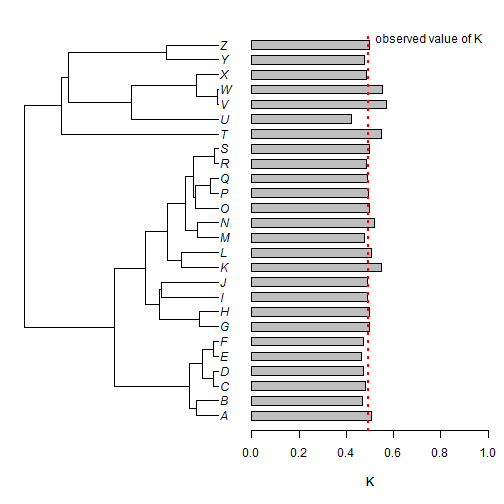Next, let's imagine that one of our observations, that of taxon `"W"` is off by 1 unit. Let's see what happens to our result:

``````x.err<-x
x.err["W"]<-x["W"]+1
K<-sapply(tips,foo,t=tree,x=x.err)
plotTree.barplot(tree,K,args.barplot=list(xlim=c(0,max(c(1,max(K)))),
xlab="K"))
abline(v=obsK,lty="dotted",col="red",lwd=2)
text(x=obsK,y=0.98*par()\$usr,"original value of K",pos=4)
abline(v=phylosig(tree,x.err),lty="dotted",col="blue",lwd=2)
text(x=phylosig(tree,x.err),y=0.5*par()\$usr,"observed value of K (with error)",pos=4)
``````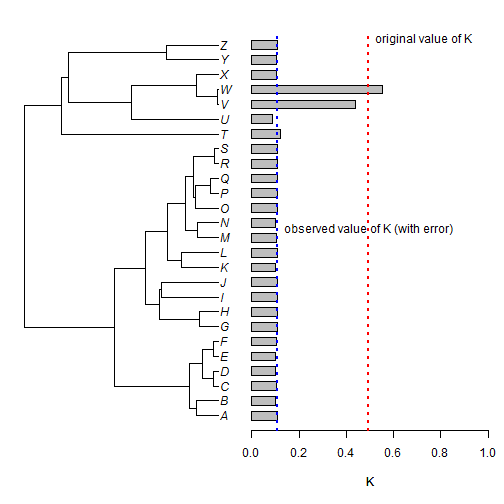You might have guessed that I didn't pick taxon `"W"` at random - it also happens to be at the end of a very short terminal edge. Let's see what happens if we do the same thing to a species that is less closely related to the others in the tree - say taxon `"T"`.

``````x.err<-x
x.err["T"]<-x["T"]+1
K<-sapply(tips,foo,t=tree,x=x.err)
plotTree.barplot(tree,K,args.barplot=list(xlim=c(0,max(c(1,max(K)))),
xlab="K"))
abline(v=obsK,lty="dotted",col="red",lwd=2)
text(x=obsK,y=0.98*par()\$usr,"original value of K",pos=4)
abline(v=phylosig(tree,x.err),lty="dotted",col="blue",lwd=2)
text(x=phylosig(tree,x.err),y=0.5*par()\$usr,
"observed value of K\n   (with error)",pos=4)
``````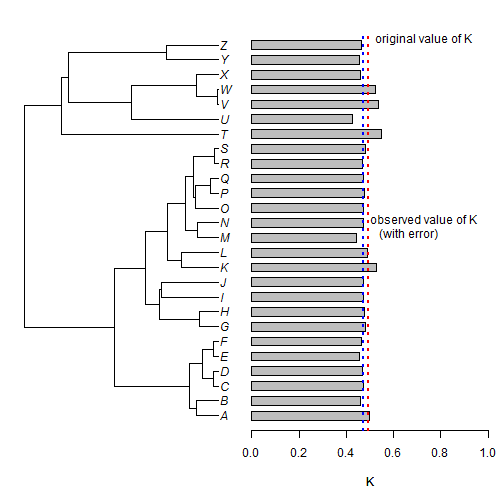Here, we can see that the same amount of error in our phenotypic value for taxon `"T"` has much less influence on the estimation of phylogenetic signal in our data.

OK, let's do the same thing now - but with the estimation of σ2 from a Brownian motion model. Here I'll use the function `evol.vcv`, because it is quite fast in this case - but we could also use `fitContinuous` from the geiger package.

``````obsSig2<-evol.vcv(tree,as.matrix(x))\$R[1,1]
obsSig2
``````
``````##  0.8490552
``````
``````foo<-function(tip,t,x){
t<-drop.tip(t,tip)
x<-x[t\$tip.label]
evol.vcv(t,as.matrix(x))\$R[1,1]
}
tips<-tree\$tip.label
sig2<-sapply(tips,foo,t=tree,x=x)
plotTree.barplot(tree,sig2,args.barplot=list(xlim=c(0,max(c(2,max(sig2)))),
xlab=expression(sigma^2)))
abline(v=obsSig2,lty="dotted",col="red",lwd=2)
text(x=obsSig2,y=0.98*par()\$usr,
expression(paste("observed value of ",sigma^2)),pos=4)
``````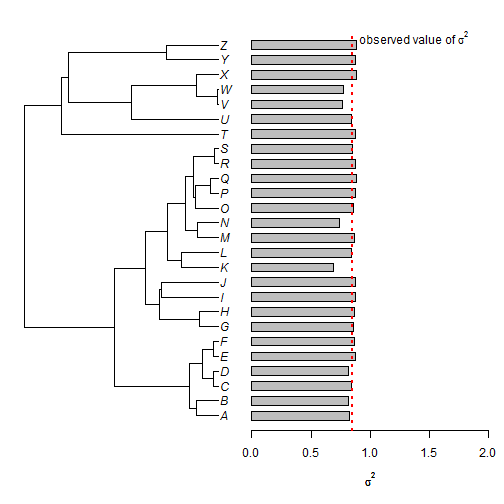Now with error:

``````x.err<-x
x.err["W"]<-x["W"]+1
sig2<-sapply(tips,foo,t=tree,x=x.err)
plotTree.barplot(tree,sig2,args.barplot=list(xlim=c(0,max(c(9,max(sig2)))),
xlab=expression(sigma^2),
main="effect of error in taxon W\n(when each taxon is excluded)",
cex.main=1))
abline(v=obsSig2,lty="dotted",col="red",lwd=2)
abline(v=evol.vcv(tree,as.matrix(x.err))\$R[1,1],lty="dotted",col="blue",lwd=2)
legend(x=4.5,y=0.98*par()\$usr,c(expression(paste("original ",sigma^2)),
expression(paste("observed ",sigma^2))),lty=rep("dotted",2),
lwd=rep(2,2),col=c("red","blue"),bty="n")
``````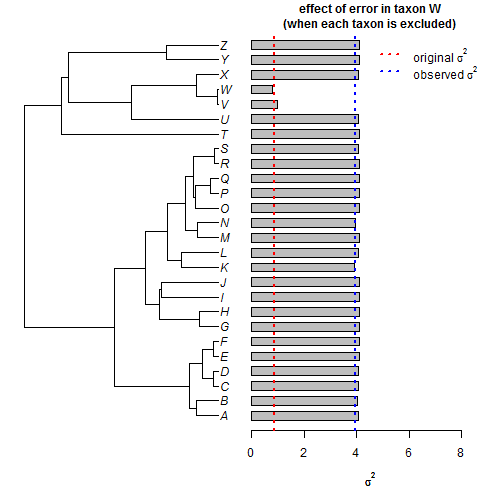``````x.err<-x
x.err["T"]<-x["T"]+1
sig2<-sapply(tips,foo,t=tree,x=x.err)
plotTree.barplot(tree,sig2,args.barplot=list(xlim=c(0,max(c(2,max(sig2)))),
xlab=expression(sigma^2),
main="effect of error in taxon T\n(when each taxon is excluded)",
cex.main=1))
abline(v=obsSig2,lty="dotted",col="red",lwd=2)
abline(v=evol.vcv(tree,as.matrix(x.err))\$R[1,1],lty="dotted",col="blue",lwd=2)
legend(x=1,y=0.98*par()\$usr,c(expression(paste("original ",sigma^2)),
expression(paste("observed ",sigma^2))),lty=rep("dotted",2),
lwd=rep(2,2),col=c("red","blue"),bty="n")
``````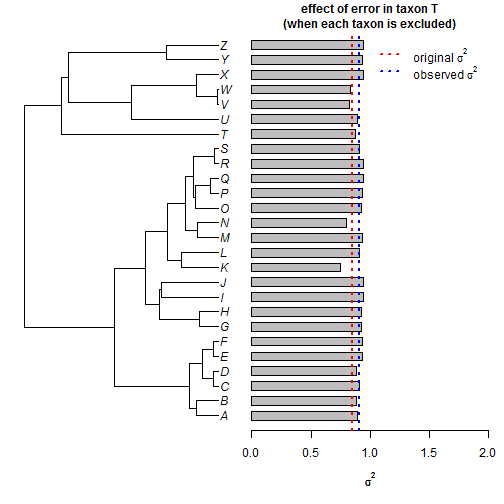As before, the weight of taxon `"W"`, and it's sister taxon `"V"`, is disproportionately high - in this case to increase the estimated value of σ2

I think there is more we could do with this type of visualization - including by dropping pairs of tips, for instance - but this is where I'll leave it for this evening.

The data for this exercise were simulated as follows:

``````tree<-pbtree(n=26,tip.label=LETTERS,scale=1)
x<-fastBM(tree)
``````

## Monday, August 28, 2017

### Confidence intervals on ancestral states under an `"EB"` model

A few days ago I posted on reconstructing ancestral states for internal nodes of the tree under a so-caled “EB” model of evolutionary change. Today, the original poster asked me the following:

“Muchas gracias Dr. Liam, ya calculé los estados ancestrales bajo el modelo EB y tambien logré graficarlos. Si yo quisera además calcular los intervalos de confianza para cada nodo (como lo hace la función `fastAnc`) podria ser posible en `anc.ML`?.”

(In other words, can we also compute variances &/or 95% CIs for states obtained under this model.)

Indeed we can.

One way to go about this would be to use the theoretically property of Maximum Likelihood estimation that tells us that the negative inverse curvature of the likelihood surface around the optimum should give us the variance-covariance matrix of our estimates. (This is what `anc.ML` does for the Brownian model.)

I have now implemented this in phytools & the update can be obtained by installing phytools from GitHub using devtools as I have shown previously.

``````library(phytools)
packageVersion("phytools")
``````
``````##  '0.6.23'
``````
``````tree
``````
``````##
## Phylogenetic tree with 100 tips and 99 internal nodes.
##
## Tip labels:
##  t29, t43, t44, t68, t75, t86, ...
##
## Rooted; includes branch lengths.
``````
``````x
``````
``````##          t29          t43          t44          t68          t75
##  0.438496287  0.472141210  0.508929413  0.476404420  0.500218520
##          t86          t87          t56          t57          t21
##  0.442388038  0.412293060  0.325040293  0.375942650  0.420729607
##          t32          t33          t71          t72          t18
##  0.144507151  0.074422614  0.188952192  0.203205398  0.156567264
##           t9          t30          t48          t49          t37
##  0.228356432  0.201488956  0.150009612  0.084055077  0.151670548
##          t23          t24           t7           t5          t88
##  0.120957147  0.276016226  0.289390081  0.286052945  0.285526927
##          t89           t1          t58          t59          t84
##  0.269553017  0.338785228 -0.638255514 -0.631750846 -0.655941964
##          t85          t16          t65          t66          t95
## -0.640326625 -0.817037017 -0.685259524 -0.663680995 -0.659381902
##          t96          t90          t79          t22          t17
## -0.676857787 -0.669947136 -0.673772868 -0.617029153 -0.709658998
##          t82          t83           t8          t10          t19
## -0.696291418 -0.688863550 -0.978188152 -0.876536606 -0.744679735
##          t20           t4          t50          t51          t67
## -0.782603431 -0.766160613 -0.779978124 -0.763065919 -0.877586463
##          t93          t94          t91          t92          t27
## -0.839376372 -0.853901636 -0.636389151 -0.643581270 -0.652135509
##          t28           t6          t42          t80          t81
## -0.657921272 -0.964749242 -0.994739494 -0.971873925 -0.970999109
##          t41          t52          t53          t62          t78
## -1.047142667 -1.030997961 -1.042163333 -1.037583807 -1.022104291
##          t99         t100          t34          t35          t45
## -1.025954239 -1.012997986 -1.047053377 -0.987320158 -1.086046548
##          t46          t36          t38          t39          t54
## -1.156241374 -1.120119673 -1.075409445 -1.036747839 -0.663847989
##          t55          t97          t98          t47          t31
## -0.698365634 -0.405954828 -0.414290095 -0.383287275 -0.344969991
##          t11          t13          t76          t77          t73
## -0.370850161 -1.095050380 -1.037155643 -1.045283871 -0.952923639
##          t74           t2           t3          t14          t63
## -1.001848791  0.141608367  0.061594482  0.313000952  0.091721162
##          t64          t69          t70          t40          t60
##  0.102012849  0.068840893  0.072838839  0.060826002  0.001699522
##          t61          t26          t25          t15          t12
##  0.065996008  0.018760874  0.174547937  0.060346979  0.097391382
``````
``````fitEB<-anc.ML(tree,x,model="EB",CI=TRUE)
fitEB
``````
``````## Ancestral character estimates using anc.ML under a(n) EB model:
##       101       102       103       104       105       106       107
## -0.149645  0.303437  0.456207  0.462999  0.483923  0.463864  0.461351
##       108       109       110       111       112       113       114
##  0.441226  0.269318  0.254532  0.256832  0.341497  0.349491  0.134272
##       115       116       117       118       119       120       121
##  0.243730  0.188746  0.195718  0.237630  0.205890  0.170694  0.146584
##       122       123       124       125       126       127       128
##  0.128694  0.246328  0.247085  0.241900  0.216083  0.277122 -0.169551
##       129       130       131       132       133       134       135
## -0.682232 -0.681542 -0.688835 -0.691968 -0.647491 -0.639588 -0.648064
##       136       137       138       139       140       141       142
## -0.671030 -0.674188 -0.669582 -0.670976 -0.669138 -0.668707 -0.680070
##       143       144       145       146       147       148       149
## -0.786477 -0.812172 -0.799949 -0.794589 -0.800530 -0.764398 -0.694839
##       150       151       152       153       154       155       156
## -0.807828 -0.807780 -0.792406 -0.773237 -0.812107 -0.785678 -0.851186
##       157       158       159       160       161       162       163
## -0.847010 -0.688502 -0.640400 -0.664724 -0.917186 -0.977880 -0.972587
##       164       165       166       167       168       169       170
## -0.811511 -1.049309 -1.042513 -1.039931 -1.054948 -1.054235 -1.032098
##       171       172       173       174       175       176       177
## -1.025175 -1.019691 -1.054352 -1.043612 -1.100283 -1.067161 -1.064348
##       178       179       180       181       182       183       184
## -0.683323 -0.392338 -0.378467 -0.391821 -0.409841 -1.036606 -1.013423
##       185       186       187       188       189       190       191
## -1.037257 -0.983257  0.044576  0.095546  0.121583  0.166058  0.101472
##       192       193       194       195       196       197       198
##  0.106063  0.072185  0.094356  0.088166  0.084205  0.070487  0.052460
##       199
##  0.039961
##
## Lower & upper 95% CIs:
##         lower     upper
## 101 -0.484549  0.185260
## 102  0.150788  0.456086
## 103  0.395288  0.517126
## 104  0.416758  0.509240
## 105  0.452711  0.515135
## 106  0.444131  0.483597
## 107  0.444389  0.478314
## 108  0.427067  0.455385
## 109  0.172091  0.366544
## 110  0.169963  0.339102
## 111  0.185330  0.328334
## 112  0.285735  0.397259
## 113  0.322108  0.376874
## 114  0.089298  0.179247
## 115  0.162741  0.324718
## 116  0.123418  0.254074
## 117  0.174228  0.217207
## 118  0.172010  0.303250
## 119  0.143025  0.268756
## 120  0.126576  0.214812
## 121  0.112322  0.180846
## 122  0.100888  0.156500
## 123  0.185768  0.306887
## 124  0.186754  0.307416
## 125  0.181730  0.302070
## 126  0.162583  0.269584
## 127  0.261368  0.292875
## 128 -0.495176  0.156074
## 129 -0.910645 -0.453818
## 130 -0.844286 -0.518798
## 131 -0.740054 -0.637615
## 132 -0.742939 -0.640997
## 133 -0.679460 -0.615522
## 134 -0.664303 -0.614873
## 135 -0.664110 -0.632018
## 136 -0.715139 -0.626921
## 137 -0.697979 -0.650397
## 138 -0.713671 -0.625492
## 139 -0.689660 -0.652293
## 140 -0.678525 -0.659750
## 141 -0.676891 -0.660522
## 142 -0.826145 -0.533995
## 143 -0.902477 -0.670476
## 144 -0.890940 -0.733404
## 145 -0.874341 -0.725557
## 146 -0.867521 -0.721656
## 147 -0.849000 -0.752061
## 148 -0.817266 -0.711531
## 149 -0.712354 -0.677324
## 150 -0.855059 -0.760597
## 151 -0.855107 -0.760453
## 152 -0.844016 -0.740795
## 153 -0.849995 -0.696478
## 154 -0.847427 -0.776787
## 155 -0.812435 -0.758921
## 156 -0.873687 -0.828686
## 157 -0.856265 -0.837755
## 158 -0.756244 -0.620759
## 159 -0.651030 -0.629770
## 160 -0.714983 -0.614465
## 161 -0.996478 -0.837893
## 162 -1.009814 -0.945947
## 163 -0.990476 -0.954697
## 164 -0.928385 -0.694638
## 165 -1.084168 -1.014449
## 166 -1.070385 -1.014641
## 167 -1.064767 -1.015095
## 168 -1.083221 -1.026674
## 169 -1.081097 -1.027373
## 170 -1.055198 -1.008998
## 171 -1.043372 -1.006979
## 172 -1.025135 -1.014247
## 173 -1.081238 -1.027466
## 174 -1.073893 -1.013330
## 175 -1.128991 -1.071575
## 176 -1.096649 -1.037674
## 177 -1.094056 -1.034639
## 178 -0.712126 -0.654520
## 179 -0.463166 -0.321509
## 180 -0.423042 -0.333892
## 181 -0.422389 -0.361253
## 182 -0.415565 -0.404117
## 183 -1.106863 -0.966350
## 184 -1.050163 -0.976684
## 185 -1.056929 -1.017585
## 186 -1.003989 -0.962524
## 187 -0.205126  0.294277
## 188 -0.025216  0.216309
## 189  0.055321  0.187845
## 190  0.104204  0.227912
## 191  0.075894  0.127050
## 192  0.047246  0.164880
## 193  0.048985  0.095385
## 194  0.042760  0.145952
## 195  0.039358  0.136973
## 196  0.041458  0.126953
## 197  0.029344  0.111630
## 198  0.019881  0.085039
## 199  0.015198  0.064724
##
## Fitted model parameters & likelihood:
##   sigma^2         r   logLik
##  0.449798 -4.169326 423.7552
##
## R thinks it has found the ML solution.
``````

Unfortunately, although estimating the ancestral states with this method is reasonably fast, computing the Hessian matrix is most definitely not as it involves numerically differentiating a very high dimensional function.

Using the curvature of the likelihood surface (or the 'Hessian' matrix) is known to be overly liberal for small sample sizes. This means that estimated variances or confidence intervals obtained in this way will tend to be too small or too narrow.

In this particular case, we can test this as we know the simulated ancestral states for our data.

First, let's plot them:

``````library(plotrix)
plotCI(a,fitEB\$ace,pch=21,pt.bg="grey",cex=1.5,ui=fitEB\$CI95[,2],li=fitEB\$CI95,
xlab="true ancestral states",
ylab="estimates states (& CIs) under EB model")
lines(range(x),range(x),lwd=1,col="red",lty="dashed")
``````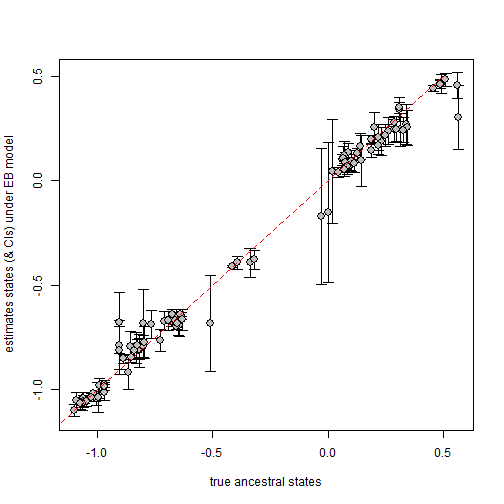We can also compute which fraction of the true ancestral states are found within the 95% CIs of the corresponding nodes. This fraction should be more or less 0.95, no?

``````mean(((a>=fitEB\$CI95[,1])+(a<=fitEB\$CI95[,2]))==2)
``````
``````##  0.7373737
``````

(Note that this operation takes advantage of the fact that, in R, mathematical operations on logical values treats them as 0s and 1s.)

We see that this quantity is far smaller than we'd like it to be - suggesting, just as we feared, that CIs based on the Hessian will be too small. (Note that I believe this is also true of `ace` in ape as described here).

An alternative solution that I believe may be valid would be to use our fitted value of r to transform the tree and then send this transformed tree to `fastAnc`. `fastAnc` does not use the Hessian matrix to compute CIs, but an analytical solution given by Rohlf (2001).

Here's what that might look like:

``````obj<-fastAnc(phytools:::ebTree(tree,r=fitEB\$r),x=x,CI=TRUE)
mean(((a>=obj\$CI95[,1])+(a<=obj\$CI95[,2]))==2)
``````
``````##  0.9090909
``````

We would need to undertake an experiment to figure out if this method gives us the right SEs and CIs, but they seem closer than those obtained from the Hessian.